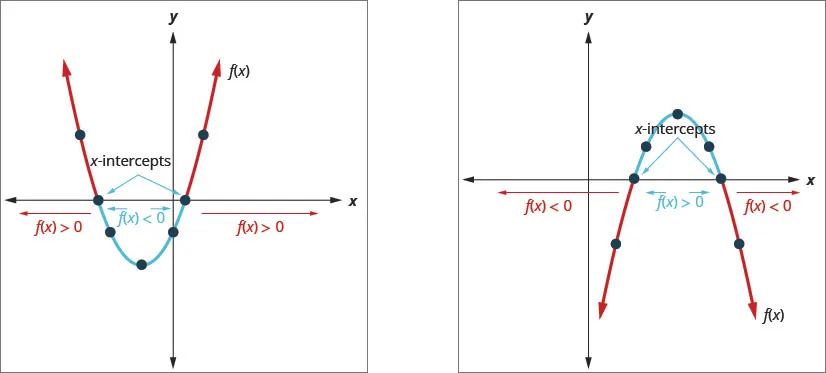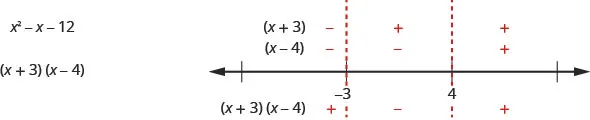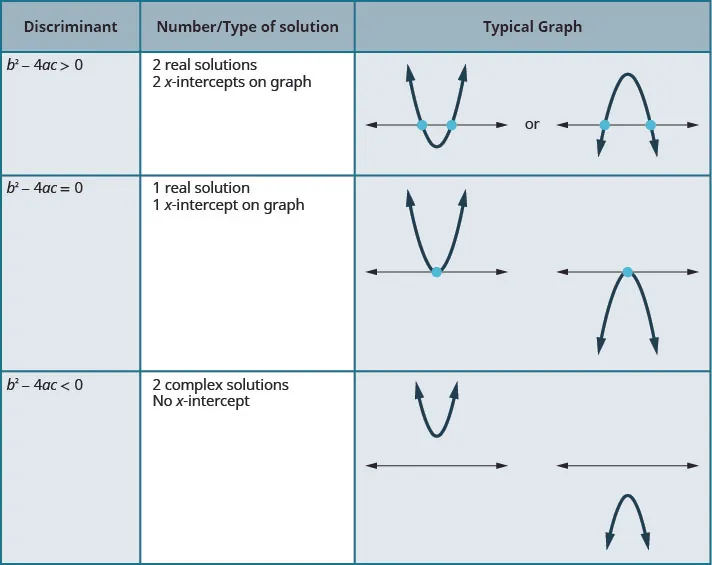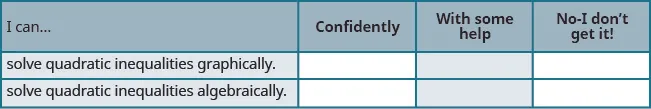Intermediate Algebra

### Learning Objectives

By the end of this section, you will be able to:

### Be Prepared 9.8

Before you get started, take this readiness quiz.

1. Solve: $2x−3=0.2x−3=0.$
If you missed this problem, review Example 2.2.
2. Solve: $2y2+y=152y2+y=15$.
If you missed this problem, review Example 6.45.
3. Solve $1x2+2x−8>01x2+2x−8>0$
If you missed this problem, review Example 7.56.

We have learned how to solve linear inequalities and rational inequalities previously. Some of the techniques we used to solve them were the same and some were different.

We will now learn to solve inequalities that have a quadratic expression. We will use some of the techniques from solving linear and rational inequalities as well as quadratic equations.

We will solve quadratic inequalities two ways—both graphically and algebraically.

A quadratic equation is in standard form when written as ax2 + bx + c = 0. If we replace the equal sign with an inequality sign, we have a quadratic inequality in standard form.

The standard form of a quadratic inequality is written:

$ax2+bx+c<0ax2+bx+c≤0 ax2+bx+c>0ax2+bx+c≥0ax2+bx+c<0ax2+bx+c≤0 ax2+bx+c>0ax2+bx+c≥0$

The graph of a quadratic function f(x) = ax2 + bx + c = 0 is a parabola. When we ask when is ax2 + bx + c < 0, we are asking when is f(x) < 0. We want to know when the parabola is below the x-axis.

When we ask when is ax2 + bx + c > 0, we are asking when is f(x) > 0. We want to know when the parabola is above the y-axis.### Example 9.64

#### How to Solve a Quadratic Inequality Graphically

Solve $x2−6x+8<0x2−6x+8<0$ graphically. Write the solution in interval notation.

### Try It 9.127

Solve $x2+2x−8<0x2+2x−8<0$ graphically and write the solution in interval notation.

### Try It 9.128

Solve $x2−8x+12≥0x2−8x+12≥0$ graphically and write the solution in interval notation.

We list the steps to take to solve a quadratic inequality graphically.

### How To

#### Solve a quadratic inequality graphically.

1. Step 1. Write the quadratic inequality in standard form.
2. Step 2. Graph the function $f(x)=ax2+bx+c.f(x)=ax2+bx+c.$
3. Step 3. Determine the solution from the graph.

In the last example, the parabola opened upward and in the next example, it opens downward. In both cases, we are looking for the part of the parabola that is below the x-axis but note how the position of the parabola affects the solution.

### Example 9.65

Solve $−x2−8x−12≤0−x2−8x−12≤0$ graphically. Write the solution in interval notation.

### Try It 9.129

Solve $−x2−6x−5>0−x2−6x−5>0$ graphically and write the solution in interval notation.

### Try It 9.130

Solve $−x2+10x−16≤0−x2+10x−16≤0$ graphically and write the solution in interval notation.

The algebraic method we will use is very similar to the method we used to solve rational inequalities. We will find the critical points for the inequality, which will be the solutions to the related quadratic equation. Remember a polynomial expression can change signs only where the expression is zero.

We will use the critical points to divide the number line into intervals and then determine whether the quadratic expression willl be postive or negative in the interval. We then determine the solution for the inequality.

### Example 9.66

#### How To Solve Quadratic Inequalities Algebraically

Solve $x2−x−12≥0x2−x−12≥0$ algebraically. Write the solution in interval notation.

### Try It 9.131

Solve $x2+2x−8≥0x2+2x−8≥0$ algebraically. Write the solution in interval notation.

### Try It 9.132

Solve $x2−2x−15≤0x2−2x−15≤0$ algebraically. Write the solution in interval notation.

In this example, since the expression $x2−x−12x2−x−12$ factors nicely, we can also find the sign in each interval much like we did when we solved rational inequalities. We find the sign of each of the factors, and then the sign of the product. Our number line would like this:The result is the same as we found using the other method.

We summarize the steps here.

### How To

#### Solve a quadratic inequality algebraically.

1. Step 1. Write the quadratic inequality in standard form.
2. Step 2. Determine the critical points—the solutions to the related quadratic equation.
3. Step 3. Use the critical points to divide the number line into intervals.
4. Step 4. Above the number line show the sign of each quadratic expression using test points from each interval substituted into the original inequality.
5. Step 5. Determine the intervals where the inequality is correct. Write the solution in interval notation.

### Example 9.67

Solve $x2+6x−7≥0x2+6x−7≥0$ algebraically. Write the solution in interval notation.

### Try It 9.133

Solve $−x2+2x+1≥0−x2+2x+1≥0$ algebraically. Write the solution in interval notation.

### Try It 9.134

Solve $−x2+8x−14<0−x2+8x−14<0$ algebraically. Write the solution in interval notation.

The solutions of the quadratic inequalities in each of the previous examples, were either an interval or the union of two intervals. This resulted from the fact that, in each case we found two solutions to the corresponding quadratic equation ax2 + bx + c = 0. These two solutions then gave us either the two x-intercepts for the graph or the two critical points to divide the number line into intervals.

This correlates to our previous discussion of the number and type of solutions to a quadratic equation using the discriminant.

For a quadratic equation of the form ax2 + bx + c = 0, $a≠0.a≠0.$The last row of the table shows us when the parabolas never intersect the x-axis. Using the Quadratic Formula to solve the quadratic equation, the radicand is a negative. We get two complex solutions.

In the next example, the quadratic inequality solutions will result from the solution of the quadratic equation being complex.

### Example 9.68

Solve, writing any solution in interval notation:

$x2−3x+4>0x2−3x+4>0$ $x2−3x+4≤0x2−3x+4≤0$

### Try It 9.135

Solve and write any solution in interval notation:
$−x2+2x−4≤0−x2+2x−4≤0$ $−x2+2x−4≥0−x2+2x−4≥0$

### Try It 9.136

Solve and write any solution in interval notation:
$x2+3x+3<0x2+3x+3<0$ $x2+3x+3>0x2+3x+3>0$

### Section 9.8 Exercises

#### Practice Makes Perfect

In the following exercises, solve graphically and write the solution in interval notation.

363.

$x 2 + 6 x + 5 > 0 x 2 + 6 x + 5 > 0$

364.

$x 2 + 4 x − 12 < 0 x 2 + 4 x − 12 < 0$

365.

$x 2 + 4 x + 3 ≤ 0 x 2 + 4 x + 3 ≤ 0$

366.

$x 2 − 6 x + 8 ≥ 0 x 2 − 6 x + 8 ≥ 0$

367.

$− x 2 − 3 x + 18 ≤ 0 − x 2 − 3 x + 18 ≤ 0$

368.

$− x 2 + 2 x + 24 < 0 − x 2 + 2 x + 24 < 0$

369.

$− x 2 + x + 12 ≥ 0 − x 2 + x + 12 ≥ 0$

370.

$− x 2 + 2 x + 15 > 0 − x 2 + 2 x + 15 > 0$

In the following exercises, solve each inequality algebraically and write any solution in interval notation.

371.

$x 2 + 3 x − 4 ≥ 0 x 2 + 3 x − 4 ≥ 0$

372.

$x 2 + x − 6 ≤ 0 x 2 + x − 6 ≤ 0$

373.

$x 2 − 7 x + 10 < 0 x 2 − 7 x + 10 < 0$

374.

$x 2 − 4 x + 3 > 0 x 2 − 4 x + 3 > 0$

375.

$x 2 + 8 x > − 15 x 2 + 8 x > − 15$

376.

$x 2 + 8 x < − 12 x 2 + 8 x < − 12$

377.

$x 2 − 4 x + 2 ≤ 0 x 2 − 4 x + 2 ≤ 0$

378.

$− x 2 + 8 x − 11 < 0 − x 2 + 8 x − 11 < 0$

379.

$x 2 − 10 x > − 19 x 2 − 10 x > − 19$

380.

$x 2 + 6 x < − 3 x 2 + 6 x < − 3$

381.

$−6 x 2 + 19 x − 10 ≥ 0 −6 x 2 + 19 x − 10 ≥ 0$

382.

$−3 x 2 − 4 x + 4 ≤ 0 −3 x 2 − 4 x + 4 ≤ 0$

383.

$−2 x 2 + 7 x + 4 ≥ 0 −2 x 2 + 7 x + 4 ≥ 0$

384.

$2 x 2 + 5 x − 12 > 0 2 x 2 + 5 x − 12 > 0$

385.

$x 2 + 3 x + 5 > 0 x 2 + 3 x + 5 > 0$

386.

$x 2 − 3 x + 6 ≤ 0 x 2 − 3 x + 6 ≤ 0$

387.

$− x 2 + x − 7 > 0 − x 2 + x − 7 > 0$

388.

$− x 2 − 4 x − 5 < 0 − x 2 − 4 x − 5 < 0$

389.

$−2 x 2 + 8 x − 10 < 0 −2 x 2 + 8 x − 10 < 0$

390.

$− x 2 + 2 x − 7 ≥ 0 − x 2 + 2 x − 7 ≥ 0$

#### Writing Exercises

391.

Explain critical points and how they are used to solve quadratic inequalities algebraically.

392.

Solve $x2+2x≥8x2+2x≥8$ both graphically and algebraically. Which method do you prefer, and why?

393.

Describe the steps needed to solve a quadratic inequality graphically.

394.

Describe the steps needed to solve a quadratic inequality algebraically.

#### Self Check

After completing the exercises, use this checklist to evaluate your mastery of the objectives of this section.On a scale of 1-10, how would you rate your mastery of this section in light of your responses on the checklist? How can you improve this?

Order a print copy

As an Amazon Associate we earn from qualifying purchases.